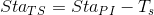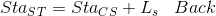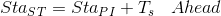### 4. Stationing

Referring to Figure E-7, curve endpoint stations are determined using Equations E-11 through E-15.Equation E-11Equation E-12Equation E-13Equation E-14Equation E-15

As with a regular horizontal curve, a station equation exists at the point where the curve system exits onto the tangent at the ST.